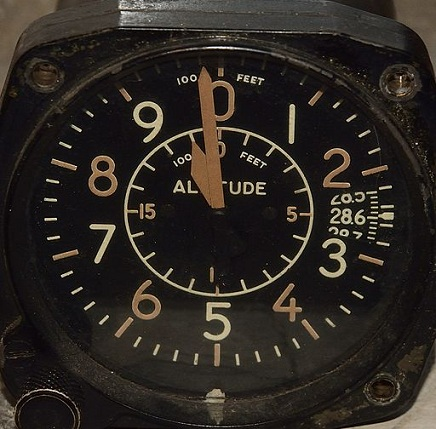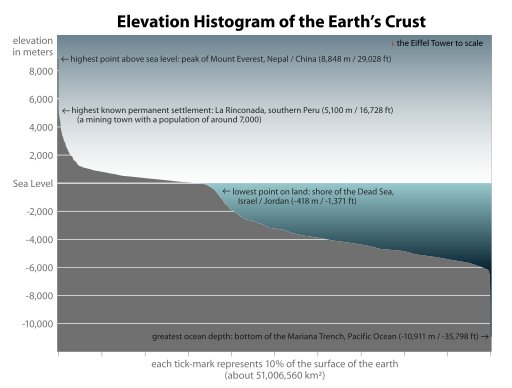# Difference Between Altitude and Elevation

## Main Difference – Altitude vs. Elevation

The terms altitude and elevation both refer to a height. The two terms have somewhat similar meanings, although there is a difference in where and how the two terms are used. The main difference between altitude and elevation is that the word altitude is used to describe the vertical distance between an object and a reference point whereas elevation is used to describe the height of a place above the sea level.

## What is Altitude

Altitude is the height of an object above a reference point or level. The reference level could be defined to be anything, although mean sea level (MSL) or above ground level (AGL) are commonly used. For example, we use the term “altitude” to refer to how high an airplane is flying. This altitude is measured using a device called an altimeter. An altimeter actually a barometer, measuring pressure. However, the higher up above the sea level you are, the less is the pressure you are going to experience (This is because at higher places, the weight of the air pushing down on you is less). Therefore, a barometer can be calibrated to give the height of a point above sea level.An altimeter from an old aeroplane

## What is Elevation

The term “elevation” is used to refer to the height of a place above the mean sea level. The image below shows a diagram showing how much of the Earth is at various altitudes:A diagram showing how much of The Earth is at various elevationsAs mentioned before, at higher elevations, the air is “thinner”. i.e. it is less dense because there is less air pushing down on it. This means that the air pressure as well as the amount of oxygen is less at higher elevations and has important implications for humans. For instance, some people suffer high-altitude sickness (note that although the word altitude is used rather than elevation, in this case, the term generally refers to the height of a place above sea level) where they experience dizziness and shortness of breath.

## Difference Between altitude and Elevation

### What it Means

Altitude refers to the height of an object above a given point.

Elevation refers to the height of a place above the mean sea level.

Image Courtesy:

“Fighter aircraft altimeter” by Łukasz Karolewski (Dozymetr) (Own work) [CC BY-SA 3.0], via Wikimedia Commons

“Earth elevation histogram” by Citynoise at en.wikipedia [public domain], via Wikimedia Commons

“The sign at an elevation of 8000 feet in the San Bernardino Mountains.” by Scottthezombie (Own work) [CC BY-SA 3.0], via Wikimedia Commons